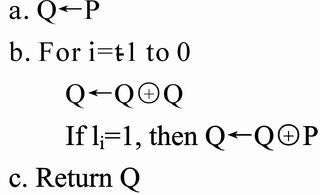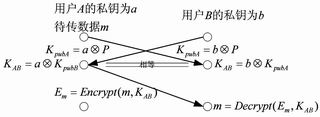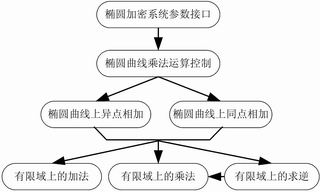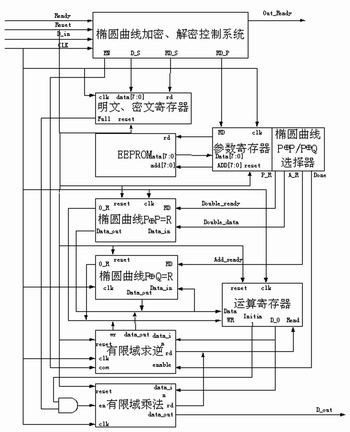# Hardware implementation of elliptic curve encryption

Abstract: Elliptic curve encryption is a public key system that can provide the highest bit strength among all currently known public key cryptosystems. When implementing an elliptic curve encryption system in FPGA, the multiplication and inversion operations in the finite field of polynomials based on GF (2) are two major difficulties. This paper provides a structure of FPGA implementation of elliptic curve encryption, focusing on the implementation of multiplication and inversion operations in the finite field of polynomials based on GF (2), and comparing the performance with the software implementation.

Security of encryption From the point of view of number theory, any public key cryptosystem is based on an NP (unmanageable problem), that is, for a specific problem, there is no way to find a polynomial time algorithm to solve the problem. The general algorithms for solving such problems are exponential time or sub-exponential time. For example, the commonly used RSA algorithm is based on the difficulty of factoring large integer factors. After nearly thirty years of research, although there is no polynomial time algorithm for the RSA algorithm, a sub-exponential time algorithm can be found. At present, the key length must be greater than 1024 bits to ensure the security of information transmission, and the elliptic curve encryption system (EllipTIc Curve Cryptosystemâ€”ECC) is a public key system that can provide the highest bit strength (Strength-Per-Bit) among all known public key cryptosystems. Only 160 keys are needed to achieve 1024-bit RSA algorithm The level of security provided. It is based on the discrete logarithm problem (ECDLP) in a point group on an elliptic curve on a finite field, and many cryptographic experts consider it to be exponentially difficult. Therefore, for the elliptic curve encryption system, from the perspective of calculation amount, processing speed, storage space and communication bandwidth, the elliptic curve encryption system has great advantages. The public key encryption algorithm standard P1363 that IEEE has formulated is based on the ECC algorithm. Now the cryptography community generally believes that it will replace RSA as a general public key cryptography algorithm, which has become a research hotspot and a promising research direction.Figure 1 Point algorithm implementationFigure 2 Key and data exchangeFigure 3 Elliptic curve encryption system structure diagramFig.4 Block diagram of FPGA circuit module of elliptic curve encryption systemFigure 5 Verification system structure

Elliptic curve encryption system Elliptic curve The introduction of Non-supersingular elliptic curve Weierstrass equation E: Y2 + XY = X3 + aX2 + c where a, câˆˆGF (2k), c â‰  0. In order to simplify the calculation in the future, introduce z to make X = x / z; Y = y / z, then the elliptic curve equation is E: y2z + xyz = x3 + ax2z + cz3, and define (x, y, z) = Î» ( x, y, z). It can be seen that when z â‰  0, (X, Y) corresponds to (x, y, z), when z = 0 can be understood as going to infinity along the y axis, defined as the point O at infinity. Then all the points on the elliptic curve plus the set of infinity points constitute an Abel group, O is the unit element (zero element). Two point operations are defined on the elliptic curve E: point operation and point operation.
1) The point operation on an elliptic curve is defined as: Let P = (x1, y1, 1) âˆˆ E, Q = (x2, y2, 1) âˆˆ E, -P = (x1, y1 + x1, 1), when Q â‰  -P when PQ = (x3, y3, z3) then when P â‰  Q:
Where A = (x2z1 + x1), B = (y2z1 + y1), C = A + B, D = A2 (A + a2z1) z1BC
When P = Q:
among them
2) The point operation on the elliptic curve is defined as: Let P = (x1, y1, 1) âˆˆ E, (ltlt-1 ... l0) 2 is the binary representation of the integer l, lP = PPAP = Q and Q E.
Using the above point operation, the point algorithm is shown in Figure 1. Define l = logpQ. If the period of P is large, it is relatively easy to find Q with l and P, but it is difficult to find l with P and Q. This is ECDLP. Elliptic curve encryption is based on this problem. on.
Encryption system In the Diffe-Hellman public key system system, the specific elliptic curve, the point P on the curve and the periodic prime N of the P are public information.
To communicate with A and B, first get the elliptic curve E, point P and prime number N. Then user A uses the randomly selected integer a in [1, N-1] as a private key, and A sends KpubA = aP as his public key to user B. At the same time, B will randomly select [1, N-1] The selected integer b is used as the private key, and KpubB = bP is sent to A as its own public key. A and B each multiply their own private key by the public key passed from each other to obtain KAB, thus completing the key exchange process. When user A needs to transmit the data m to be transmitted to user B, A uses m and KAB to generate Em. When user B gets Em, he uses the KAB generated by the key exchange process and the encrypted data Em obtained from user A to generate Data m. See Figure 2.

Elliptic encryption system implementation In the elliptical encryption systems that have been put into use so far, most of the key lengths are relatively short, generally concentrated in 30 to 60 bits. This is because when the software is implemented, due to the limitation of the software execution rate, the key The rate of the ellipse encryption system with a relatively large length (â‰¥160) will not meet the requirements for use. At the same time, in hardware implementation, elliptic encryption systems with large key lengths will consume a lot of hardware resources. With the in-depth study of elliptic encryption algorithms and the rapid development of programmable logic devices, it is already a possible choice to use programmable logic devices to implement an elliptical encryption system. The following will introduce an implementation scheme, which is implemented in software and hardware respectively.
According to the requirements of the above elliptical encryption system, the structure diagram of the encryption system in FIG. 3 is designed, in which the parameter interface of the ellipse encryption system obtains the basic parameters of the ellipse related to encryption, such as the private key, elliptic curve, and a given point on the elliptic curve. The elliptic curve multiplication control part is mainly responsible for how to calculate the multiplication result. It will call a large number of PP and PQ to realize the multiplication function; and PP and PQ get the result through the call of finite field addition, multiplication and inversion.
Software model verification The main purpose of software implementation is to establish a verification model for hardware implementation. The structure of the entire software is shown in Figure 3. During the implementation of the software verification system, the addition on the finite field is an XOR operation. Multiplication and inversion on the finite field are the key points, and resource consumption during hardware implementation must be considered in advance, and efficient algorithms are needed. In this system, the particularity brought by the complex domain GF ((2n) m) is used, which can efficiently and quickly realize multiplication and inversion operations.
* Multiplication on GF (2n): A (y) & TImes; B (y) = C (Y) modQ (y), Q (y) is the reduced polynomial. Commonly used are: Paar-Rosner multiplier, Mastrovito multiplier, Massey-Omura multiplier, Hasan-Bhargava multiplier, etc. Here are two options:
1) When n is relatively small, it can be realized by the table look-up method, set Ï‰ as the primitive root of Q (y) = 0, then F2n = {0, Ï‰, AÏ‰2n-1}, use the table look-up method to obtain the number of A and B levels a, b, C order c = a + b, use look-up table method again to get C from c. This method is used in this system to achieve multiplication on GF (2n).
2) When n is relatively large, resource consumption is too large to bear using the look-up table method. C = Z & TImes; B (when n is relatively large), Z is a matrix determined by A (y) and Q (y), among them:

* Multiplication of compound finite fields: Taking GF ((24) 2) as an example, the multiplication of GF (28) can be constructed by multiplication and addition on GF (24). The primitive polynomial of subfield GF (24) is Q (y) = y4 + y + 1, the primitive polynomial of the second subfield is R (z) = z3 + z + Ï‰14, where Ï‰ is GF (24) The base element satisfies Q (Ï‰) = 0. The multiplication of two elements in the field [a0 + a1z] & TImes; [b0 + b1z] can be expressed as:

In this way, the multiplication of GF ((24) 2) on the composite field can be obtained by the mathematical operation of the finite field on GF (24).
* Inverse operation of compound finite field: The inverse of element A in compound finite field GF ((2n) m) is:
Among them, it can be observed that Ar belongs to the element in the subfield GF (2n), and the value of (Ar) -1 can be easily obtained.
FPGA hardware implementation Software-based implementation method development time is short, but its encryption speed is relatively slow, which hinders the practicality of elliptic curve encryption. The FPGA method combines the flexibility of software and the security of hardware, and provides superior speed than the software method. Compared with traditional ASIC implementation, programmable devices are more suitable for cryptography applications due to their high flexibility. field.
Based on the software model, we optimized the model for the characteristics of the FPGA hardware. According to the requirements of the elliptic curve encryption algorithm, the encryption system is designed modularly. Each module independently completes its own function, and the modules exchange data and control each other to achieve the encryption function. Fig. 4 is a block diagram of a circuit module implemented by an elliptic curve encryption system FPGA.
Among them, the elliptic curve encryption control system module is the core of the entire system. When Ready is True, the system reads in the initial data and controls the RAM to store the initial data. During the calculation process, the module performs a control loop on the selector according to the data source, performs PP = R and PQ = R operations to obtain the final result, and then outputs the result through the Out_Ready signal; the selector module according to the instructions provided by the control system module Control PP = R module and PQ = R module, and provide corresponding real-time data flow; PP = R module and PQ = R module use timing control to add and multiply operations on the finite field to find the point on the elliptic curve The addition operation will directly affect the speed performance of the entire system. Therefore, a reasonable input and output data flow must be designed for the addition and multiplication operations on the finite field to achieve a high efficiency operation rate. Various memory modules store the initial value of the system, the intermediate value during the operation, and the system operation result according to different instructions.
Based on the above factors, we chose the VirtexII device from XILINX, ISE 4.1 as the development platform, and VHDL as the development language. Because the calculation amount of the 168-bit elliptic curve encryption algorithm is relatively large, when FPGA is implemented, wiring is a factor worth considering. The selection of FPGA devices should take into account the routing resources. The routing resources provided by the Virtex series are relatively abundant. The performance index obtained after simulation on Modelsim is: the initial setup time is required for the first encryption or decryption under the 40MHz clock drive, and the output of the plain text or cipher text takes about 2ms, and the output of the subsequent plain text or cipher text is about 25Mbps. It can be seen that this is a relatively high rate and can be applied to many occasions.
Application system verification After the ellipse encryption hardware is implemented, it must be verified in the actual system. We specially constructed a serial encryption experiment board for verification. The structure of the entire verification system is shown in Figure 5. After actual system verification, it proves that the hardware implementation of the above elliptical encryption system is successful.

Conclusion Public key cryptosystems are usually used for key management, key exchange, digital signature, and authentication, etc., where less information is involved due to their high computational and time complexity. At present, the old algorithms such as DES and RSA are still widely used. The update of the algorithm can not only make the original cryptographic users obtain better performance, but also can make IC cards, mobile phones and other fields that are difficult to implement cryptographic algorithms available. Cryptography technology to ensure information security.
The elliptic curve cryptosystem (ECC) is attracting industry attention with its shorter keys and theoretically higher strength, and the hardware implementation of the elliptic curve cryptosystem (ECC) will also be a focus in public key cryptosystems . Although this article has laid a good foundation for future work, there is still a lot of work to be done in the following aspects. The first is the development of programmable logic devices. In the future, there will be devices that can provide a larger number of gates and provide a faster rate; secondly, the improvement of the elliptic curve cryptosystem itself; and finally, the further improvement of the hardware implementation algorithm of finite field mathematical operations. With the development of the above aspects, the hardware implementation that can provide longer keys and faster data rates will provide a faster and safer encryption system for national economic and social development.

PM Stepper Motor

Pm Stepper Motor,Square Flange Stepper Motor,High Precision Stepper Motor,Permanent Magnet Stepper Motor

Changzhou Sherry International Trading Co., Ltd. , https://www.sherry-motor.com